Math Courses / Course / Chapter

# Matrices: Notation, Order, Equal Matrices & Math Operations

Rashid Nuzha, Yuanxin (Amy) Yang Alcocer, Kathryn Boddie
• Author
Rashid Nuzha

Rashid holds a Postgraduate Diploma in Professional Studies in Education from The Open University-United Kingdom and a Bachelor of Science in Physics and Mathematics. He has 10+ years of experience from theory to practice in educational leadership and management.

• Instructor
Yuanxin (Amy) Yang Alcocer

Amy has a master's degree in secondary education and has been teaching math for over 9 years. Amy has worked with students at all levels from those with special needs to those that are gifted.

• Expert Contributor
Kathryn Boddie

Kathryn has taught high school or university mathematics for over 10 years. She has a Ph.D. in Applied Mathematics from the University of Wisconsin-Milwaukee, an M.S. in Mathematics from Florida State University, and a B.S. in Mathematics from the University of Wisconsin-Madison.

Understand what a matrix is in math, how proper matrix notation is written, and what is matrix order. Using examples of matrices, learn about equal matrices and matrix math operations. Updated: 12/23/2021

Show
• FAQs
• Activities

In the following examples, students will apply their knowledge of addition, subtraction, and multiplication of matrices to determine various sums, differences, and products. Some problems will use only one operation whereas others will combine operations.

## Examples

For the following matrices,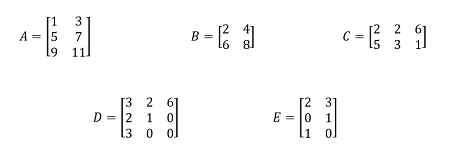determine the following, if they exist.

1) A + E

2) B - C

3) DE

4) ED

5) (A + E)B

6) (A - E)C

## Solutions

1) Since A and E are the same size (they are both 3x2 matrices), we can add them by adding their corresponding elements.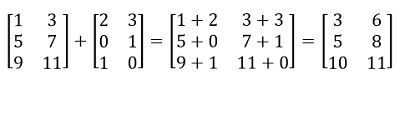2) We cannot calculate B - C because the matrices are not the same size.

3) We can calculate DE because the number of columns of D matches the number of rows of E.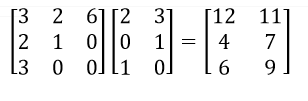Remember to multiply a row of D by a column of E. For example, the first entry in the product is found by multiplying the first row of D by the first column of E to get 3(2) + 2(0) + 6(1) = 6 + 0 + 6 = 12.

4) We cannot calculate ED because the number of columns of E (2) does not match the number of rows of D (3). Note that this means that the order of multiplication matters - we could calculate DE but not ED.

5) We found A + E in number 1. We can multiply (A + E) and B because the number of columns of (A + E) matches the number of rows of B.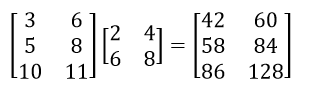6) We can subtract E from A because the matrices are the same size. Then, we can multiply (A - E) by C because the number of columns of (A - E) matches the number of rows of C.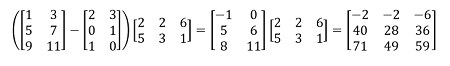#### What is a 2x3 matrix?

A 2x3 or 2 by 3 matrix is a matrix that has 2 rows and 3 columns. Every matrix is referred to by two numbers: the first is the number of rows and the second is the number of columns. Also, 2x3 is called the matrix order.

#### What happens when two matrices are equal?

When two matrices are equal, they become the same matrix and so referring to any of the two is the same as referring to the other one. The same logic applies when referring to n number of equal matrices; any one of them can represent them all.

#### How do you notate a matrix?

Use an upper case letter to notate a matrix. For example writing matrix A is correct while writing matrix a is incorrect. Matrix elements are notated with the lower case version of the matrix name with subscript numbers denoting their row and column position.

#### How do you know if matrices are equal?

For two matrices to be called equal, all of their elements that share the same notations must be equal. This is equal to saying that the element a1,1 from matrix A must be equal to element b1,1 from matrix B and so forth for all corresponding elements from both martices.

A matrix is an arrangement of numbers (in general, real numbers) in rows and columns where each number is called a matrix element. Every matrix is named after its number of rows and columns; for example, a 2x3 matrix is a matrix with two rows and three columns. In matrix math, all matrices are written as the number of rows x the number of columns. This row x column value is called the order of the matrix.

To unlock this lesson you must be a Study.com Member.

## Matrices

Matrices are math objects where the numbers are organized into a nice rectangular array of rows and columns. They are useful to learn because we can turn a system of equations into matrix form. We can manipulate matrices more easily than a collection of equations. To get you on the right track, we will look at matrices in this video lesson, their proper notation, their allowed math operations, and how to tell when two matrices are equal to each other.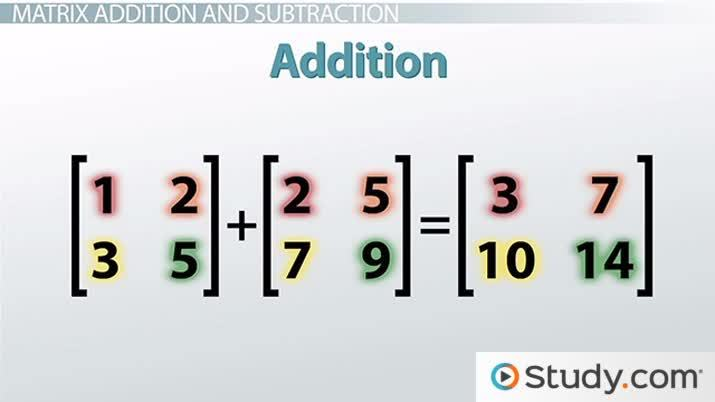An error occurred trying to load this video.

Try refreshing the page, or contact customer support.

Coming up next: How to Solve Inverse Matrices

### You're on a roll. Keep up the good work!

Replay
Your next lesson will play in 10 seconds
• 0:03 Matrices
• 0:31 Notation
• 1:16 Equal Matrices
• 3:02 Matrix Multiplication
• 6:06 Lesson Summary
Save Timeline
Autoplay
Autoplay
Speed Speed

Every matrix is made up of matrix elements that are represented with matrix notation as lower case letters with subscripts to specify their position in the matrix. For example, {eq}a_2,_3 {/eq} denotes an element in row 2 and column 3, and {eq}a_3,_1 {/eq} denotes an element in row 3 and column 1.

To unlock this lesson you must be a Study.com Member.

Two matrices are considered equal matrices when all elements that carry the same notation in both matrices are equal. It is clear that this definition includes that both matrices must be of the same order . When all matrices in a group of matrices are equal any matrix of the group can represent the whole group.

For example, if A 3x1 and B 1x3 matrices are as follows:

A= {eq}\begin{pmatrix} 5\\ 9\\ 8\\ \end{pmatrix} {/eq}

and

B={eq}\begin{pmatrix} 5& 9& 8\\ \end{pmatrix} {/eq}

A and B are not equal matrices because A is 3x1 order and B is 1x3 order

Example 2:

Find t using the equal matrices A and B below.

A={eq}\begin{pmatrix} 94& 305& -2\\ \end{pmatrix} {/eq}

B={eq}\begin{pmatrix} 94& 305& t\\ \end{pmatrix} {/eq}

Solution: compare the elements in both matrices to find that {eq}a_1,_3=b_1,_3=t=-2 {/eq}

To unlock this lesson you must be a Study.com Member.

To add matrices, add elements that have the same notations from the matrices. Also, keep in mind that it is not possible to add matrices unless they are of the same order.

Example 1:

A={eq}\begin{pmatrix} 7 & 9\\ 2 & 4\\ -6& -8\\ \end{pmatrix} {/eq}

and B={eq}\begin{pmatrix} -9 & 4\\ -2 & 14\\ 0& 7\\ \end{pmatrix} {/eq}

C={eq}\begin{pmatrix} 3 & -8\\ 6 & -23\\ 7& -3\\ \end{pmatrix} {/eq}

Solution:

A+B+C is possible because all matrices have the same order of 3 rows and 2 columns: 3x2.

so:

To unlock this lesson you must be a Study.com Member.

Subtracting matrices is similar to adding them. Matrices must be of the same order to be able to subtract them.

Example 1:

Subtract C=A-B where A and B are:

A={eq}\begin{pmatrix} -7& -4& 4 & 8 & 0 \\ 21& 15 & -8& 7& 15\\ 4& 7& -7& 46& 32 \\ \end{pmatrix} {/eq}

B={eq}\begin{pmatrix} -1& 4& -9 & 4 & 5 \\ 30& -9 & 2& 8& 7\\ -21& 7& 0& 53& -4 \\ \end{pmatrix} {/eq}

Solution:

To unlock this lesson you must be a Study.com Member.

Find the multiplication of matrix A by matrix B, C=A*B. What order is C?

A={eq}\begin{pmatrix} 1& -5& 2\\ -4& 0 & 3\\ \end{pmatrix} {/eq}

B={eq}\begin{pmatrix} 1 & -1& 1 & 2\\ 0& 2& 4& -2\\ 2& 3& -1& 0\\ \end{pmatrix} {/eq}

Solution:

To find matrix C, here are examples of how to calculate matrix C elements:

{eq}C_1,_1=a_1,_1*b_1,_1+a_1,_2*b_2,_1+a_1,_3*b_3,_1 {/eq}

{eq}C_1,_1=1*1+(-5)*0+2*2 {/eq}

{eq}C_1,_1=5 {/eq}

and

{eq}C_2,_3=a_2,_1*b_1,_3+a_2,_2*b_2,_3+a_2,_3*b_3,_3 {/eq}

{eq}C_2,_3=-4*1+0*4+3*(-1) {/eq}

{eq}C_2,_3=-7 {/eq}

So, matrix C can be written as:

C=

To unlock this lesson you must be a Study.com Member.

Matrix division in itself is not a defined matrix operation. However, we can multiply a matrix by the inverse of a matrix. Remember that 2 divided by 3 is the same as saying 2 is multiplied by the inverse of 3, written as {eq}2/3=2*(1/3)=2*3^{-1} {/eq}

Similarly, matrix A divided by B is equal to saying A multiplied by the inverse of B, written as {eq}A/B=A*(1/B)=A*B^{-1} {/eq}

So, the division process is turned to finding a multiplication by the inverse of a matrix.

Example:

Find A/B where matrices A and B are as follows:

A={eq}\begin{pmatrix} a_1,_1& a_1,_2\\ a_2,_1& a_2,_2\\ \end{pmatrix} {/eq}

and B={eq}\begin{pmatrix} b_1,_1& b_1,_2\\ b_2,_1& b_2,_2\\ \end{pmatrix} {/eq}

Solution:

A/B is equal to saying {eq}A*B^{-1} {/eq}

Now, to find the inverse of matrix B, {eq}B^{-1} {/eq}, remember to first find the determinant of matrix B and then multiply it by a different arrangement of matrix B:

{eq}d=b_1,_1*b_2,_2-b_1,_2*b_2,_1 {/eq}

then

{eq}B^{-1}=(1/d)*\begin{pmatrix} b_2,_2& -b_1,_2\\ -b_2,_1& b_1,_1\\ \end{pmatrix} {/eq}

To unlock this lesson you must be a Study.com Member.

A matrix is an arrangement of numbers in rows and columns. Matrices are used in many scientific fields, such as computer science, mathematics, economics, engineering, and physics. Matrices are made up of elements that are referred to by two notations, the first refers to the row number and the second refers to the column number. Two matrices are equal when all elements that share the same notations from both matrices are equal and the matrices have the same order.

In adding or subtracting matrices, simply add or subtract elements that have the same notations and keep in mind that only matrices that are of the same order can be added or subtracted.

In multiplying two matrices, a certain method is followed where the order of the matrices matters. Dividing two matrices A/B can be done by multiplying A by the inverse of the second matrix B.

To unlock this lesson you must be a Study.com Member.

## Notation

To begin, we look at the proper notation for matrices. Look at this properly written matrix: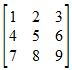Our numbers are arranged in neat rows and columns. Surrounding our matrix are square brackets. These square brackets tell us that this particular group of numbers belongs together in one matrix. We can also label our matrix by calling it matrix A. If we have labeled our matrix, we can either write out the matrix with all the numbers in it or we can write it using our label surrounded by square brackets. Our matrices are also described by their size. This particular matrix has three rows and three columns, so we can also call it a 3x3 matrix. A matrix with four rows and two columns is a 4x2 matrix.

## Equal Matrices

Okay, now that we know what proper matrix notation looks like, let's talk about when two matrices are equal to each other. You know how when two numbers are the same, they are the identical number, like when you have 2 and 2. Well, matrices are similar, but there is just a bit more involved. When two matrices are the same, all the numbers must be the same in the same positions and the matrices must both be the same size. For example, these two matrices are the same: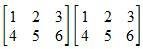They are both the same size and all the numbers are the same in the same spots. They are both 2x3 matrices.

Take a look at these matrices. Are these the same?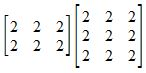They are not equal matrices because they are different sizes. Just because two matrices have all the same numbers does not mean they are equal. Their sizes must also be the same.

Let's cover the kinds of math operations we can do. We can do addition and subtraction. Matrix addition and subtraction is the same as number addition and subtraction. We add or subtract number by number. Because we have to match our number to number, our two matrices must be the same size, like this: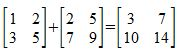We matched the numbers together. We matched the number in the first row and first column in the first matrix to the number in the first row and first column in the second matrix and so on. We can't add or subtract two matrices that are different sizes. We can however add or subtract the same number to all the numbers in a matrix.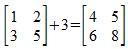We perform subtraction in the same way as addition. The two matrices must be the same size and we subtract number to number, matching the location of the numbers together. We can also subtract the same number from all the numbers in a matrix.

## Matrix Multiplication

The last math operation we can do is matrix multiplication. There is no such thing as matrix division. Matrix multiplication is more complicated than number multiplication. You can easily multiply 3 and 5 to make 15. But with matrices, when we multiply two matrices together, we have to use a combination of multiplication and addition. Also, the number of columns in the first matrix must match the number of rows in the second matrix. So, we can multiply a 1x3 matrix with a 3x2 matrix, but we can't multiply a 1x3 matrix with a 2x3 matrix. And unlike numbers where the order of multiplication doesn't matter, with matrices, which matrix comes first matters.

Let's look at how we multiply two matrices together: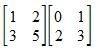Video Transcript

## Matrices

Matrices are math objects where the numbers are organized into a nice rectangular array of rows and columns. They are useful to learn because we can turn a system of equations into matrix form. We can manipulate matrices more easily than a collection of equations. To get you on the right track, we will look at matrices in this video lesson, their proper notation, their allowed math operations, and how to tell when two matrices are equal to each other.

## Notation

To begin, we look at the proper notation for matrices. Look at this properly written matrix:Our numbers are arranged in neat rows and columns. Surrounding our matrix are square brackets. These square brackets tell us that this particular group of numbers belongs together in one matrix. We can also label our matrix by calling it matrix A. If we have labeled our matrix, we can either write out the matrix with all the numbers in it or we can write it using our label surrounded by square brackets. Our matrices are also described by their size. This particular matrix has three rows and three columns, so we can also call it a 3x3 matrix. A matrix with four rows and two columns is a 4x2 matrix.

## Equal Matrices

Okay, now that we know what proper matrix notation looks like, let's talk about when two matrices are equal to each other. You know how when two numbers are the same, they are the identical number, like when you have 2 and 2. Well, matrices are similar, but there is just a bit more involved. When two matrices are the same, all the numbers must be the same in the same positions and the matrices must both be the same size. For example, these two matrices are the same:They are both the same size and all the numbers are the same in the same spots. They are both 2x3 matrices.

Take a look at these matrices. Are these the same?They are not equal matrices because they are different sizes. Just because two matrices have all the same numbers does not mean they are equal. Their sizes must also be the same.

Let's cover the kinds of math operations we can do. We can do addition and subtraction. Matrix addition and subtraction is the same as number addition and subtraction. We add or subtract number by number. Because we have to match our number to number, our two matrices must be the same size, like this:We matched the numbers together. We matched the number in the first row and first column in the first matrix to the number in the first row and first column in the second matrix and so on. We can't add or subtract two matrices that are different sizes. We can however add or subtract the same number to all the numbers in a matrix.We perform subtraction in the same way as addition. The two matrices must be the same size and we subtract number to number, matching the location of the numbers together. We can also subtract the same number from all the numbers in a matrix.

## Matrix Multiplication

The last math operation we can do is matrix multiplication. There is no such thing as matrix division. Matrix multiplication is more complicated than number multiplication. You can easily multiply 3 and 5 to make 15. But with matrices, when we multiply two matrices together, we have to use a combination of multiplication and addition. Also, the number of columns in the first matrix must match the number of rows in the second matrix. So, we can multiply a 1x3 matrix with a 3x2 matrix, but we can't multiply a 1x3 matrix with a 2x3 matrix. And unlike numbers where the order of multiplication doesn't matter, with matrices, which matrix comes first matters.

Let's look at how we multiply two matrices together:To unlock this lesson you must be a Study.com Member.

### Register to view this lesson

Are you a student or a teacher?

Back

### Resources created by teachers for teachers

Over 30,000 video lessons & teaching resources‐all in one place.Video lessonsQuizzes & WorksheetsClassroom IntegrationLesson Plans

I would definitely recommend Study.com to my colleagues. It’s like a teacher waved a magic wand and did the work for me. I feel like it’s a lifeline.

Jennifer B.
TeacherCreate an account to start this course today
Used by over 30 million students worldwide